# Python Tutorials

## Programming Basics and Math Python

 Section 1. 開始 Section 2. 極限與微分 Section 3. 極值問題 Section 4. 泰勒展開與洛比達 Section 5. 積分 Section 6. 微分方程式

## Section 1. 開始

### 主題 1. 裝置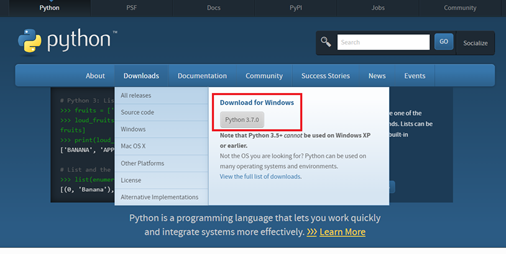Step 2. 下載軟體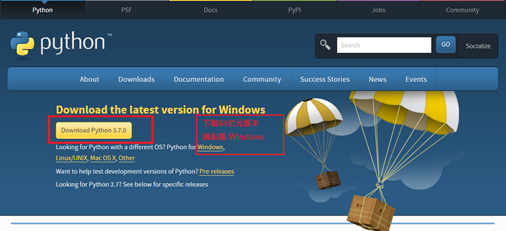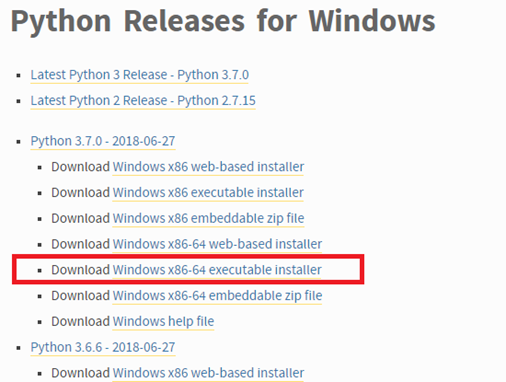Step 3. 下載完成後，啟動安裝檔。3.7版的選項，可以在裝置時就設定好環境變數。如果是初學者，請務必一步一步看清楚選項，避免事後又出現一堆設定問題。如圖 1-4指示，下方打勾可以寫入環境變數路徑(path)；如果忘了打勾，事後還是可以另外由控制台進入填寫。Step 4. 裝置完畢後，以Windows 7為例，以IDLE啟動Python (開啟Python的方法還可以透過「命令提示字元」或Power Shell。為了不讓初學者感到負擔過重，我們現階段只介紹IDLE一種，之後會以Spyder 為主)。IDLE是Python GUI(Graphical User Interface)，如圖 1-5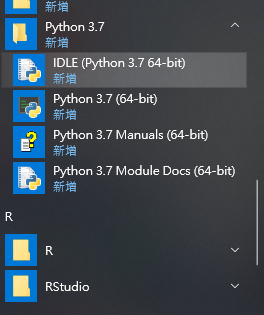>>> 1+1

>>> 2y=2
y=3
x+y### 主題 2. 基礎數學運算

(一) 四則運算
>>> 6+5                                              加
11
>>> 6-9                                               減
-3
>>> 81/9                                             除(整除)
9.0
>>> 80/9                                 除(非整除)
8.88888888888889
>>> 80//9                                除(無條件捨去)
8
>>> 5*5*5                              5的3次方=53
125
>>> 5**3                                5的3次方另一種表示方法
125

>>> 300%15                    餘數
0
>>> 300%11
3

(二) 數值型態轉換
>>> int(2.125)                 整數
2
>>> round(3.14159) 四捨五入
3.0
>>> round(3.14159, 2)
3.14
>>> round(3.14159, 3)
3.142

>>> import operator    載入比較算子模組(因下面的函數需要)（Python2 比較大小有一個函數cmp，我們用的 Python3 沒有這函數，相關功能是由模組 operator 達成。）

>>> operator.lt(2, 3)   是否2<3? lt=less than
True
>>> operator.gt(2, 3)   是否2>3? gt=greater than
False
>>> operator.eq(-2, -3)  是否-2=-3? eq=equal to
False
>>> operator.ne(2, 2)  是否 2¹2? ne=not equal to
False

>>> import math                       載入數學模組math (因下面的函數需要)
>>> math.floor(2.71828) 小於等於2.71的最大整數
2                                                                                      (數線上，邊的第1個整數)
>>> math.ceil(2.71828) 大於等於2.71的最小整數
3                                                                                      (數線上，邊的第1個整數)
>>> math.sqrt(9)                          平方根3.0
>>> abs(-123)                              絕對值 |-123|
123
>>> math.exp(2)                          自然指數 e2
7.38905609893065
>>> math.exp(1)                         自然指數 e
2.718281828459045
>>> math.log(100)       自然對數 ln(100)
4.605170185988092
>>> math.log10(100)     以10為底的對數
2.0
>>> math.pi                                             p
7.38905609893065
>>> math.factorial(5)                   5!＝1x2x3x4x5
120
>>> x=(1, 3, 5, 7, 9)
>>> max(x)                                              最大值
9
>>> min(x)                                  最小值
1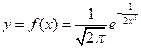，請用計算Python計算f(-1.23)=?

>>> x = -1.23
>>> y = (1/math.sqrt(2*math.pi))*math.exp(-1/(2*x*x))
>>> y
0.2866682534882691

### 主題 3 指派運算子Assignment Operator

Assignment Operator為一種運算子，用以指派給一變數或資料結構。Python 有多個指派算子。

 指派算子 範例 解釋 = x = 50 數字 50 指定給 x += x += 50 同 x = x + 50 -= x -= 50 同 x = x – 50 *= x *= 50 同 x = x * 50 /= x /= 50 同 x = x / 50 %= x %= 50 同 x = x % 50 //= x //= 50 同 x = x // 50 **= x **= 50 同 x = x ** 50 &= x &= 50 同 x = x & 50 |= x |= 50 同 x = x | 50 ^= x ^= 50 同 x = x ^ 50 <<= x <<= 50 同 x = x << 50 >>= x >>= 50 同 x = x >> 50

a = 6
x = 36
x += a  # 使用 +=
print(x)
x -= a      # 使用 -=
print(x)
x *= a                  # 使用 *=
print(x)
x //= a                 # 使用 //=
print(x)
x **= a    # 使用 **=
print(x)
x /= a      # 使用 /=
print(x)
x %= a    # 使用 %=
print(x)
x = 33
y = 88
x &= y    # 使用 &=
print(x)
x |= 9       # 使用 |=
print(x)
x ^= y      # 使用 ^=
print(x)Anaconda是一個使用Python的IDE(Integrated Development Environment)，裝置同時，也裝好Python所有設定和套件下載十分簡易。

Anaconda外觀如圖Py 4-2，右邊的Spyder就是很受歡迎的Python IDE，其他的工具也很有用。但是，Anaconda 帶入的 Python 版本不是最新的，是最新前一版。其實，這多半不是問題。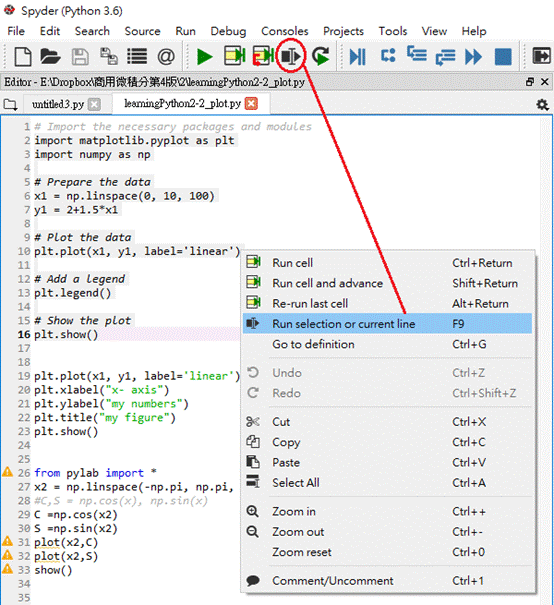IDLE的主控台無法清理，但是Spyder只需要在命令列(如圖In  的位置)鍵入cls就可以。Python 程式碼可以這樣處理

import scipy as sp
import numpy as np

A=sp.mat('[1 2 -5; 2 5 1; 2 3 8]')
B = sp.mat('[10;-8;5]')

A.I*B

np.linalg.solve(A, B)

請自行確認答案是不是
matrix([[ 25.96551724], [-11.68965517], [ -1.48275862]])

Python乃至任何程式語言都有流程控制，也就是條件式判斷True/False。流程控制用於檢查條件是否吻合，以執行下一步程式；例如，通關密語，過關為真(True)，可繼續執行下一步。可以分為「關係運算子」，「邏輯運算子」和「if…., then」等三類。依序介紹

5.1 關係運算子 relational operator

 關係運算子 意義 範例 說明 < 小於 A 大於 A>B 檢查A是否大於B >= 大於或等於 A>=B 檢查A是否大於等於B == 等於 A==B 檢查A是否等於B != 不等於 A!=B 檢查A是否不等於B

>>> print(10>9)
>>> print(10<=10)
>>> print(10 != 0)
>>> print(5== 5)

>>> X=10>9
>>> print(X)

5.2 邏輯運算子 logical operator

(1) A and B: 「A為真」且「B為真」，此事件才為真

>>> X=(10>5) and (23>15)
>>> print(X)
True

>>> X=(3.45>3) and (2>5)
>>> print(X)
False

(2) A or B: 「A為真」或「B為真」，兩者一個為真，此事件就為真
>>> X=(4>3) or (2>5)
>>> print(X)
True

(3) not():
>>> X= not(4>3)
>>> print(X)
False

>>> X= not(5<10)
>>> print(X)
True

5.3 if 敘述
if 敘述的基本語法如下
if (條件):
條件為真執行的程式

if 敘述的內容較多，讀者此時可以啟動Spyder來練習。舉例如下

if (score >= 60):
print("恭喜！")
print("你的微積分成績過關了。")

if (score>=60){
printf("恭喜！你微積分成績過關了。");
printf("抱歉！微積分明年重修囉。");
}

number = int(input(" 請輸入任何整數: "))
if number >= 1:
print(" 您輸入的是正整數 ")
print(" 您輸入的不是正整數 ")

5.4 「if….. else」的敘述

if (條件):
條件為真，執行的程式區塊
else:
條件不為真時，執行的程式區塊

if (score >= 60):
print("恭喜！你微積分成績過關了。")
else:
print("抱歉！微積分明年重修囉。")

import math
x0=input("請輸入正負1之間任意小數： ")
x=float(x0)
if (abs(x)>1):
print("錯誤！")
print("輸入數字超過邊界")
else:
y=math.log((1+x)/(1-x))
print(y)

1. 請刪除上例之範圍條件，然後將轉換改成指數函數。
2. 請將上例之範圍條件改成非負的正數(³0)，然後將轉換改成指數函數，然後輸入很大的x，和3-1的習題第五題確認一下。

5.5 「if….elif…. else」的敘述

這是一個多重判斷，寫程式時遇到多個條件的分類時，就很有用。例如，目前已經被很多大學採用的成績的等第制度。90~100是A+，85~89是A，80~84是A-，77~79是B+，73~76是B，70~72是B-，研究生的最低標準是B-。

sc=input("請輸入成績： ")
score=int(sc)
if (score >= 90):
print("A+")
elif (score >= 85):
print("A")
elif (score >= 80):
print("A-")
elif (score >= 77):
print("B+")
elif (score >= 73):
print("B")
elif (score >= 70):
print("B-")
else:
print("Failed")

5.6 巢狀的if敘述

巢狀(nested)的if敘述是指if內還有一層if

age = int(input(" 請輸入年齡:  "))
if age < 18:
print(" 您未成年 ")
print(" 您的年齡不符合本專案規範 ")
else:
if age >= 18 and age <= 60:
print(" 您符合本專案規範 ")
print(" 請填妥以下表格完成申請 ")
else:
print(" 您已經超齡 ")
print(" 您的年齡不符合本專案規範")

寫程式是不是很好玩？慢慢學，和自己課程結合，很容易的。如前所說，函數定義了一組運算，接下來我們要學習如何用Python定義函數，幫助我們計算一些複雜的公式。

1. 請設計一個可以執行以下4件事的程式：
(1) 若輸入英文大寫，則轉成小寫
(2) 若輸入英文小寫，則轉成大寫
(3) 若輸入數字，則直接輸出
(4) 其他字元，則輸出「錯誤」兩字

2. 請設計一個程式，可以執行以下階梯函數的計算：